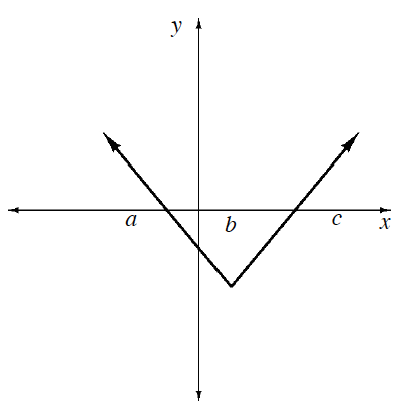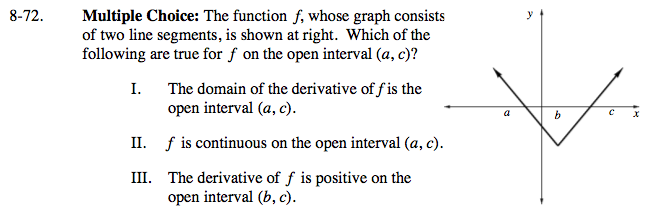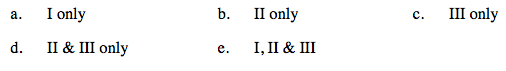### Home > CALC > Chapter 8 > Lesson 8.2.1 > Problem8-72

8-72.
1.Multiple Choice: The function f, whose graph consists of two line segments, is shown at right. Which of the following are true for f on the open interval (a, c)? Homework Help ✎

1. The domain of the derivative of f is the open interval (a, c).

2. f is continuous on the open interval (a, c).

3. The derivative of f is positive on the open interval (b, c).

1. I only

2. II only

3. III only

4. II & III only

5. I, II & IIIIs there anywhere within a < x < c in which the derivative does not exist?

Check all three conditions of continuity.

A positive derivative means that f(x) is increasing.